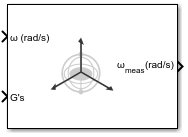# Three-axis Gyroscope

Implement three-axis gyroscope

•Libraries:
Aerospace Blockset / GNC / Navigation

## Description

The Three-Axis Gyroscope block implements a gyroscope on each of the three axes. For more information on the measured body angular rates, see Algorithms.

Optionally, to apply discretizations to the block inputs and dynamics along with nonlinearizations of the measured body angular rates, use the Saturation block.

## Limitations

• Anisoelastic bias and anisoinertial bias effects are not accounted for in this block.

• This block is not intended to model the internal dynamics of different forms of the instrument.

## Ports

### Input

expand all

Angular rates in the body-fixed axes, specified as a three-element vector, in radians per second.

Data Types: `double`

Accelerations in the body-fixed axes, specified as a three-element vector, in Gs.

Data Types: `double`

### Output

expand all

Measured angular rates from the gyroscope, returned as a three-element vector, in radians per second.

Data Types: `double`

## Parameters

expand all

To apply second-order dynamics to gyroscope readings, select this check box.

#### Programmatic Use

 Block Parameter: `dtype_g` Type: character vector Values: `'on'` | `'off'` Default: `'on'`

Natural frequency of the gyroscope, specified as a double scalar, in radians per second.

#### Programmatic Use

 Block Parameter: `w_g` Type: character vector Values: double scalar Default: `'190'`

Damping ratio of the gyroscope, specified as a double scalar.

#### Programmatic Use

 Block Parameter: `z_g` Type: character vector Values: double scalar Default: `'0.707'`

Scale factors and cross-coupling, specified as a 3-by-3 matrix, to skew the gyroscope from body axes and to scale accelerations along body axes.

#### Programmatic Use

 Block Parameter: `g_sf_cc` Type: character vector Values: 3-by-3 matrix Default: ```'[1 0 0; 0 1 0; 0 0 1]'```

Long-term biases along the gyroscope axes, specified as a three-element vector, in radians per second.

#### Programmatic Use

 Block Parameter: `g_bias` Type: character vector Values: 3-by-3 matrix Default: ```'[0 0 0]'```

Maximum change in rates due to linear acceleration, specified as a three-element vector, in radians per second per g-unit.

#### Programmatic Use

 Block Parameter: `g_sen` Type: character vector Values: three-element vector Default: ```'[0 0 0]'```

Update rate of the gyroscope, specified as a double scalar, in seconds. An update rate of 0 creates a continuous gyroscope. If the Noise on check box is selected and the update rate is 0, the block updates the noise at a rate of 0.1.

Tip

If you:

• Update this parameter value to 0 (continuous)

• Configure a fixed-step solver for the model

you must also select the Automatically handle rate transition for data transfer check box in the Solver pane. This check box enables the software to handle rate transitions correctly.

#### Programmatic Use

 Block Parameter: `g_Ts` Type: character vector Values: double scalar Default: `'0'`

To apply white noise to the gyroscope readings, select this check box.

#### Programmatic Use

 Block Parameter: `g_rand` Type: character vector Values: `'on'` | `'off'` Default: `'on'`

Scalar seeds for the Gaussian noise generator for each axis of the gyroscope, specified as a three-element vector.

#### Dependencies

To enable this parameter, select Noise on.

#### Programmatic Use

 Block Parameter: `g_seeds` Type: character vector Values: three-element vector Default: ```'[23093 23094 23095]'```

Height of the power spectral density (PSD) of the white noise for each axis of the gyroscope, specified as a three-element vector, in (rad/s)2/Hz.

#### Dependencies

To enable this parameter, select Noise on.

#### Programmatic Use

 Block Parameter: `g_pow` Type: character vector Values: three-element vector Default: ```'[0.0001 0.0001 0.0001]'```

Three minimum values and three maximum values of angular rates in each of gyroscope axes, specified as a six-element vector, in radians per second.

#### Programmatic Use

 Block Parameter: `g_sat` Type: character vector Values: six-element vector Default: ```'[-inf -inf -inf inf inf inf]'```

## Algorithms

The measured body angular rates $\left({\overline{\omega }}_{meas}\right)$ include the body angular rates $\left({\overline{\omega }}_{b}\right)$, errors, and, optionally, the discretizations and nonlinearizations of the signals:

`${\overline{\omega }}_{meas}={\overline{\omega }}_{b}×{\overline{\omega }}_{SFCC}+{\overline{\omega }}_{bias}+Gs×{\overline{\omega }}_{gsens}+noise$`

where ${\overline{\omega }}_{SFCC}$ is a 3-by-3 matrix of scaling factors on the diagonal and misalignment terms in the nondiagonal, ${\overline{\omega }}_{bias}$ are the biases, (Gs) are the Gs on the gyroscope, and ${\overline{\omega }}_{gsens}$ are the G-sensitive biases.

 Rogers, R. M., Applied Mathematics in Integrated Navigation Systems, AIAA Education Series, 2000.